Posted on October 30, 2020

# A Bayesian Perspective on Training Speed and Model Selection

TL;DR Models that train faster (with respect to the number of data points they need to fit the dataset) have a higher marginal likelihood. We leverage this to get estimators of the (log) marginal likelihood in linear models that depend on the sum of training losses obtained in an iterative updating procedure. We show an intriguing connection between these estimators and the weight assigned to features in linear regression, and find that the intuition driving these estimators also seems to hold in deep neural networks.

# Introduction

Suppose that you’re trying to build a hotdog classifier, and you have a choice between two neural networks: network A has a cross-entropy loss of 0.01 on the training set, and it reached that loss extremely quickly. Network B obtains a cross-entropy loss of 0.0001 on the training set, but it took a lot longer to get below 0.01 than Network A. You want to use the model that will be the most accurate on new, unseen possible-hotdogs: which do you pick?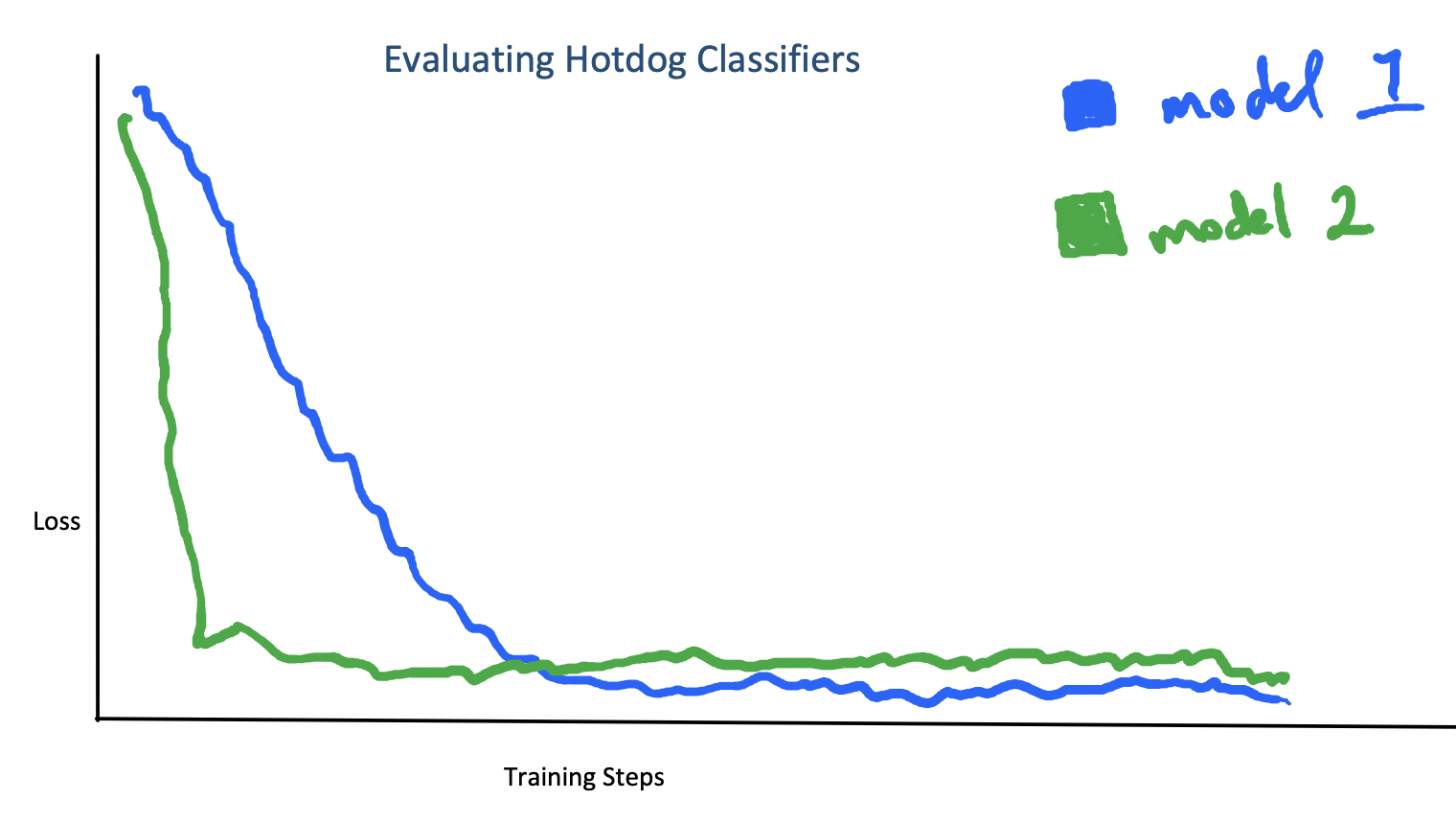A difficult model selection problem.

If you held out some training data for a validation set, then you can pick the one with better performance on the unseen data and be done. But suppose you don’t have access to a validation set. Is there still a way of justifying the intuition that the model that got a pretty low loss quickly is less likely to have overfit than the model that got an extremely low loss slowly? It turns out the answer is yes – if you’re willing to be Bayesian.

## Background on Model Selection

TL;DR

• Model selection and generalization are deeply connected
• Generalization in neural networks is not well-understood
• There are some generalization bounds that are related to training speed
• A Bayesian approach to model selection is to pick the model with the highest marginal likelihood

# Estimating the Marginal Likelihood

### Decomposing the log ML

OK, so if we want to perform model selection by maximizing the marginal likelihood, we’ll need to compute the marginal likelihood first. Observe that we can write the (log) ML as follows.

$\log P(\mathcal{D}) = \sum \log P(\mathcal{D}_i|\mathcal{D}_{<i})$

Making the dependence of the conditional $$P(D_i|D_{<i})$$ on the parameters $$\theta$$ more explicit, we get

$\log P(\mathcal{D}) = \sum \log \mathbb{E}_{P(\theta | \mathcal{D}_{<i})}[P(\mathcal{D}_i | \theta)]$

In other words: the marginal likelihood measures how well a posterior update based on one subset of the data $$\mathcal{D}_{<i}$$ is able to predict the next data point $$\mathcal{D}_i$$. We can visualize the log ML as computing the ‘area under the curve’ of posterior predictive probabilities.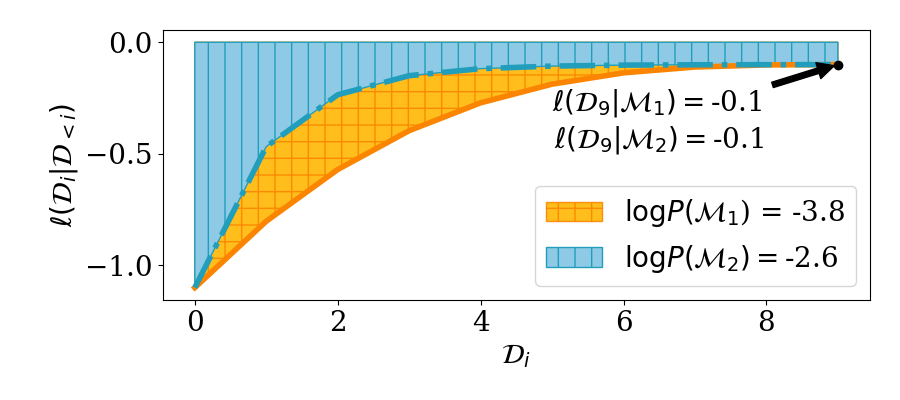### Estimator Zoo

The value $$\log \mathbb{E}[P(\mathcal{D}_i|\theta)]$$ isn’t always possible to compute exactly. However, given parameter samples from the posterior, it’s straightforward to estimate a lower bound.

$\log P(\mathcal{D}) = \sum \log \mathbb{E}_{P(\theta | \mathcal{D}_{<i})}[P(\mathcal{D}_i | \theta)] \ge \sum \mathbb{E}_{P(\theta | \mathcal{D}_{<i})}[ \log P(\mathcal{D}_i | \theta)] = L(D)$

Where the inequality follows from Jensen’s inequality. We can make this inequality tighter by averaging over multiple parameter samples before applying the logarithm to get another estimator that we’ll call $$L_k$$.

$\log P(\mathcal{D}) \geq \sum \mathbb{E}_{P(\theta | \mathcal{D}_{<i})} \; [ \log \frac{1}{k} \sum_{j=1}^k P(\mathcal{D}_i | \theta_j)] = L_k(D)$

Finally, if we have samples from the posterior predictive distribution $$P(\hat{D_i} | D_{<i})$$ and this distribution is a Gaussian, then we can use our posterior samples to estimate the parameters $$\mu, \sigma^2$$ of $$P(\cdot | D_{<i})$$ and then use those estimated parameters in the log likelihood term to obtain yet another estimator $$L_S$$.

$\log P(\mathcal{D}) = \sum_{i=1}^n \mathbb{E}[\log P(\mathcal{D}_i|\widehat{\mu}_i, \widehat{\sigma}^2_i)] = L_S(D)$

Each of these estimators has its own pros and cons: $$L$$ has an intriguing interpretation from the minimum description length framework, but can have a large bias term when used to estimate the ML. $$L_k$$ reduces this bias term, but both $$L_k$$ and $$L$$ can’t be applied to models whose likelihood is a dirac delta distribution (i.e. models with zero observation noise). $$L_S$$ works even for models with zero observation noise, but only yields an unbiased estimate of a lower bound when the posterior predictive is Gaussian (otherwise this may no longer be a lower bound).

### Estimating the log ML via Gradient Descent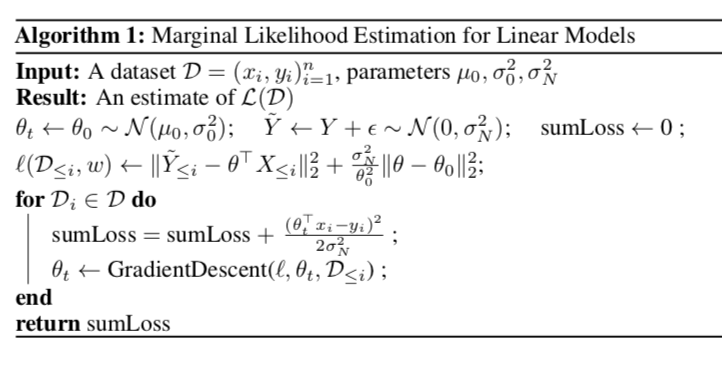TL;DR: In linear models, we can sample parameters from a prior and then run gradient descent to get a posterior sample to feed into the estimators from the last section.

### Linear model combinations and $$L(D)$$

TL;DR: if you train a linear regressor on top of predictions from Bayesian models concurrently as the models are being updated, then the model with the highest weight is the one with the highest $$L(D)$$, not the one whose final posterior is the best fit.

### Empirical Results

TL;DR: our estimators agree with the marginal likelihood on what the ‘best’ model is in a number of different settings.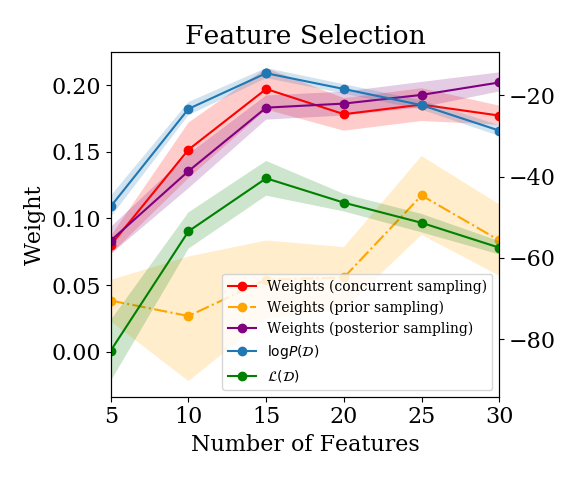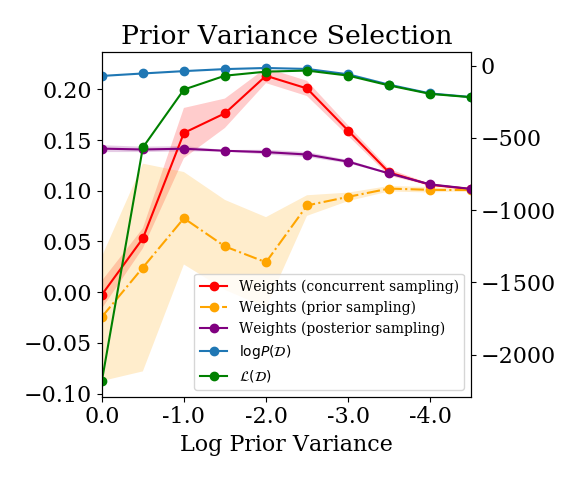Estimated log P(D) and weight assigned by a linear model combination for various model selection problems. Our estimators give models similar rankings to the log marginal likelihood.

# Connection to Deep Learning

Recall that the ML measures how well updates from one subset of the data generalize to unseen data points. This gives us an analogy between training speed, as measured by the sum of log predictive posterior likelihoods, and Bayesian model selection. Training speed in a Bayesian updating procedure is defined with respect to the number of data points needed to assign high likelihood to the rest of the training data. However, in many settings where we might want to do model selection, we don’t necessarily have exact posterior samples from a Bayesian model conditioned on increasing subsets of the data. Standard gradient-based optimization of deep neural networks (DNNs) involves iterating through the entire dataset repeatedly for several epochs, so we’ll need to consider training speed with respect to the number of gradient steps taken, rather than the number of data points seen. In this section, we’re going to take a first stab at the following question:

Does there exist a connection between a notion of training speed that captures within-training-set generalization in DNNs, and their test set generalization performance?

To apply the Bayesian intuition to neural networks requires us to map some of the concepts discussed earlier in the Bayesian setting to the risk minimization setting.

• Bayesian model $$\equiv$$ function approximator
• Posterior $$P(\theta|D) \equiv$$ Point estimate $$\theta^*$$
• $$\sum \log P(D_i|D_{<i}) \equiv \sum_{t=1}^T \ell(D_{i_t}, \theta_t)$$
• Marginal likelihood $$\equiv$$ generalization error

While there are many interesting lines of work framing stochastic gradient optimization as performing posterior sampling, in the analysis that follows we won’t be using this interpretation. Nor will we try to cast classifiers as computing unnormalized probability distributions. Instead, we’re going to focus on using minibatch SGD to measure how well model updates based on one subset of the data generalize to other subsets.

### Motivation: Minibatch Loss as a Risk Estimator

TL;DR: when you compute a minibatch gradient update, your loss on the next, disjoint minibatch gives you an idea of how well that gradient update will generalize to your test set.

### Empirical Results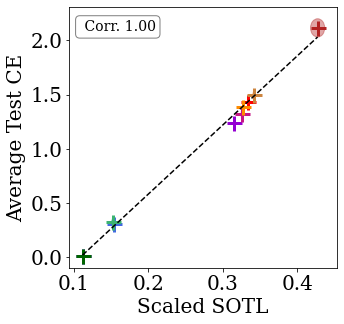Models which train faster (as measured by their sum over training losses) generalize better.
We now ask whether the connection between $$\mathcal{L}(\mathcal{D})$$ and the weight assigned to a model in a linear model combination might also apply in some form to the deep learning setting. The lemma doing the heavy lifting in the proof of this result basically says that the weight assigned to a feature in a linear regression problem depending to some extent on that feature being fairly predictive of the target (modulo a bunch of assumptions). We make a similar conjecture for the weight assigned to activations in the penultimate layer of a neural network: features which are more predictive of the targets will be assigned higher weight. Because the final-layer weights and activations are trained concurrently, we expect that the notion of how predictive a node is will be more closely aligned with the SOTL definition rather than its final ‘training loss’.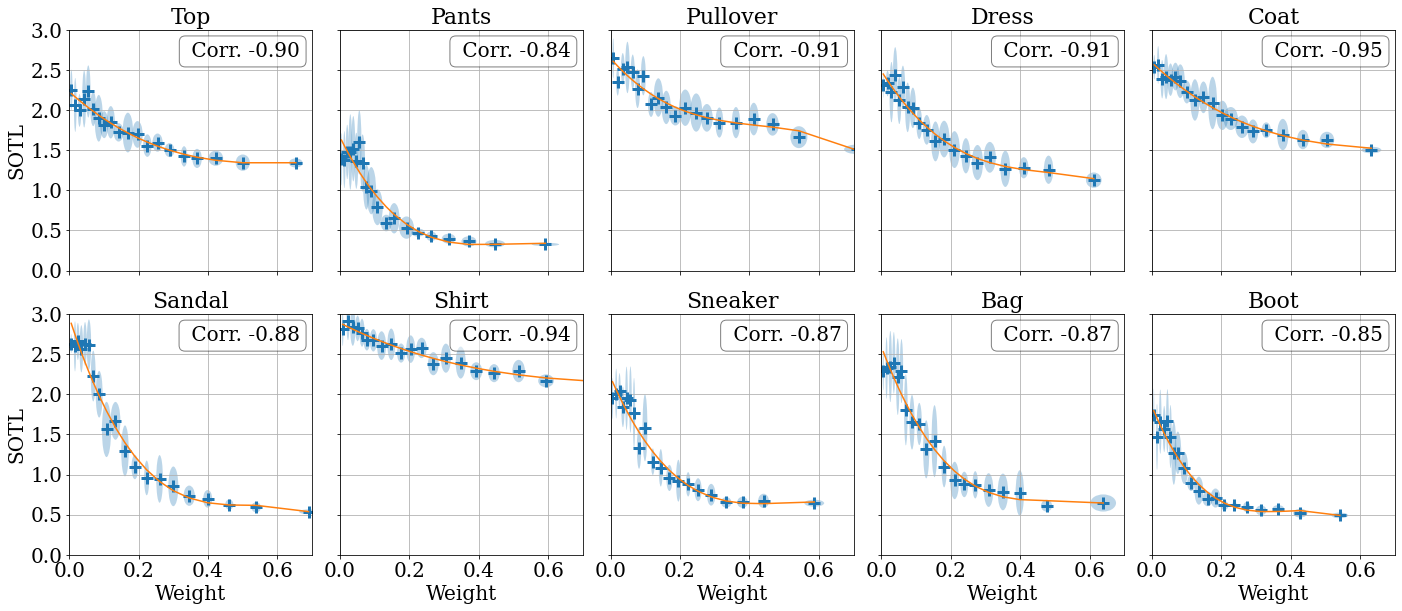Layer activations which train faster (as measured by their sum over ‘information losses’) are assigned higher weight by the final layer of a neural network.

To investigate this claim, we train a linear combination of models, and see whether the test loss correlates with the assigned model weight. We observe that SGD tends to upweight models that generalize better. This suggests that SGD may implicitly perform model selection. Interestingly, we see that this phenomenon also holds for subnetworks within a network. The above figure shows that SGD upweights sub-networks with lower SOTL. These results are far from the final word on the topic, and we believe they present an interesting direction for future work.

### Recap

The goal of this paper was to analyze the connection between Bayesian model selection and training speed in order to gain insight into how models generalize. We proposed a family of estimators of the marginal likelihood that measure a notion of training speed, and which depend only on samples from the posteriors of Bayesian models. We further showed that this quantity is equivalent to measuring how well updates based on one subset of the data generalize to other subsets, and that an analogous measure on deep neural networks also appears to empirically correlate with generalization error. Our results thus point both to a way of doing principled Bayesian model selection on families of models from which we can obtain posterior samples, and also to a direction of inquiry towards understanding generalization in complex function approximators based on optimization trajectories rather than only post-training quantities.